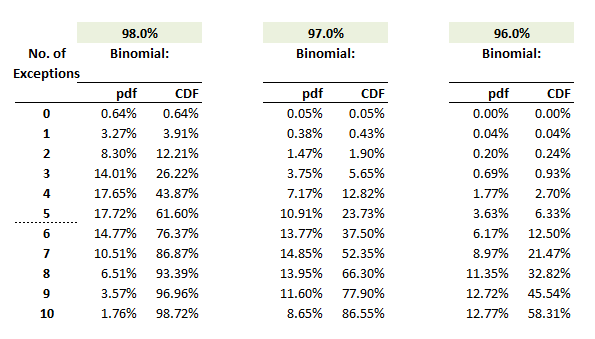# P2.T5.22.7 Value at risk (VaR) backtest

#### Nicole Seaman

##### Director of FRM Operations
Staff member
Learning objectives: Describe backtesting and exceptions and explain the importance of backtesting VaR models. Explain the significant difficulties in backtesting a VaR model. Verify a model based on exceptions or failure rates.

Questions:

22.7.1. Mary the FRM conducts a backtest of her firm's one-day 99.0% confident value at risk (VaR) model. Her backtest counts the number of exceptions over a two-year historical window that includes 500 daily observations; two years * 250 days/year = 500 days. She applies the binomial distribution (displayed in the exhibit below) given the parameters. n = 500 and p = 0.010.She selects a cutoff of nine exceptions. Consequently, she will decide the VaR model is inaccurate (aka, bad model) if she observes nine or more exceptions during the window; i.e., if X ≥ 9 → reject null). If the model is accurate, what is the probability that she commits a type I (aka, type 1) error?

a. 1.79%
b. 3.11%
b. 3.60%
d. 6.71%

22.7.2. Peter the FRM conducts a backtest of his firm's one-day 99.0% confident value at risk (VaR) model. His backtest counts the number of exceptions over a two-year historical window that includes 500 daily observations; two years * 250 days/year = 500 days. His binomial distribution assumes the parameters, n = 500 and p = 0.010. He selects a cutoff of nine exceptions. Consequently, he will decide the VaR model is inaccurate (aka, bad model) if he observes nine or more exceptions during the window; i.e., if X ≥ 9 → reject null. Otherwise, he will accept the model as accurate, because he fails to reject the null hypothesis.

While an accurate model, in this case, is described by only one confidence level (i.e., 99.0%), there are several possible inaccurate models. Below are displayed three specific inaccurate models for true coverage levels of 98.0%, 97.0%, and 96.0%.If the firm's model is inaccurate and its true coverage level is 98.0%, what is the implied probability of type II (aka, type 2) error?

a. 2.81%
b. 11.30%
c. 33.05%
d. 66.95%

22.7.3. Mary the FRM plans to conduct a backtest of her firm's one-day 99.0% confident value at risk (VaR) model. She is lucky to have much data. Her backtest counts the number of exceptions over a four-year historical window that includes 1,000 daily observations; four years * 250 days/year = 1,000 days. She will apply the binomial distribution given the parameters. n = 1,000 and p = 0.010. She observes the binomial Pr(X ≤ 4) = 2.87% and Pr(X ≥ 17) = 1 - Pr(X ≤ 16) = 2.64%. Consequently, the 95.0% acceptance (aka, nonrejection) region is given by 4<N<17, and this matches Jorion's Table 6-2.

She observes 16 exceptions and she, therefore, accepts the model as accurate. However, she subsequently realizes this is a two-sided test. But the firm is much more concerned about a VaR that is inaccurately too aggressive rather than too conservative. If she conducts a one-sided 95.0% confident test of the 99.0% VaR model, what is her decision?

a. Decide the model is inaccurate (aka, bad) because the observation is in the rejection region
b. Decide the model is accurate (aka, good) because the observation is in the acceptance region
c. Her test is invalid because the VaR confidence level is 99.0% but the confidence level for the decision rule is only 95.0%
d. Her test is invalid because she selected the wrong one-tail test; i.e., the alternative hypothesis should be Ha: p<0.010 rather than Ha: p>0.010